Register for Maths, Science, English, Reasoning Olympiad Exams Register here | Book Free Demo for Live Olympiad Classes here | Check Olympiad Exam Dates here | Buy Practice Papers for IMO, IOM, HEO, IOEL etc here | Login here to participate in all India free mock test on every Saturday

# Trigonometry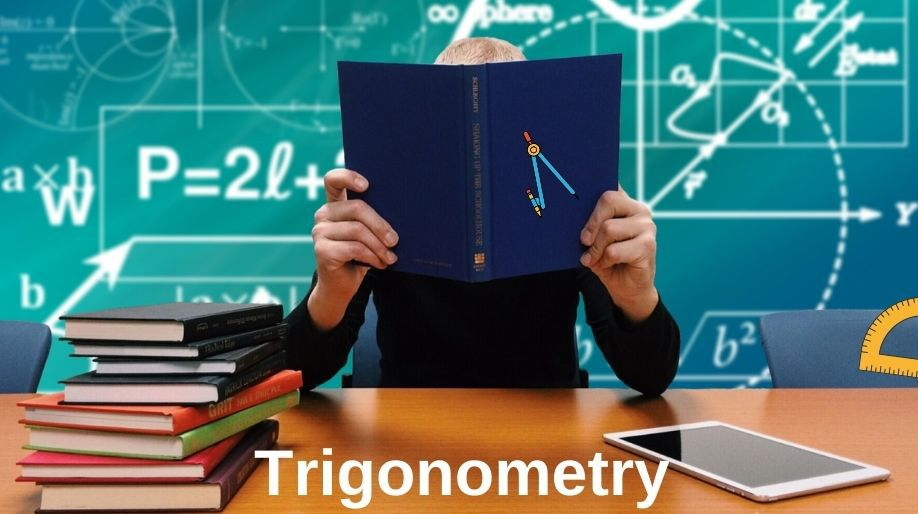According to J.F. Herbart “There is perhaps nothing which occupies the middle position of mathematics as trigonometry". From the statement itself we can guess that what a great or important role trigonometry plays in Mathematics. Let’s start from the beginning and learn how interesting it is.

Trigonometry is basically the measuring of sides, angles and other things involved in a triangle and here we will deal with right angle triangle, the angles involved and many other things.

Trigonometry is derived from Greek words “tri" means three “gon" means sides and “metron" means measurements.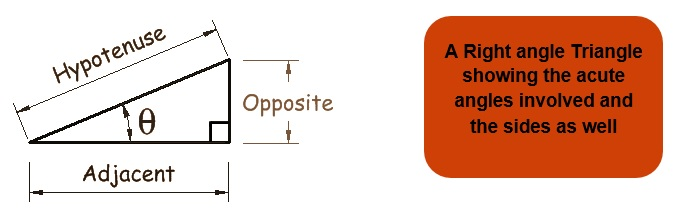Here we will study the different Trigonometric Ratios different terms identities related to Trigonometry.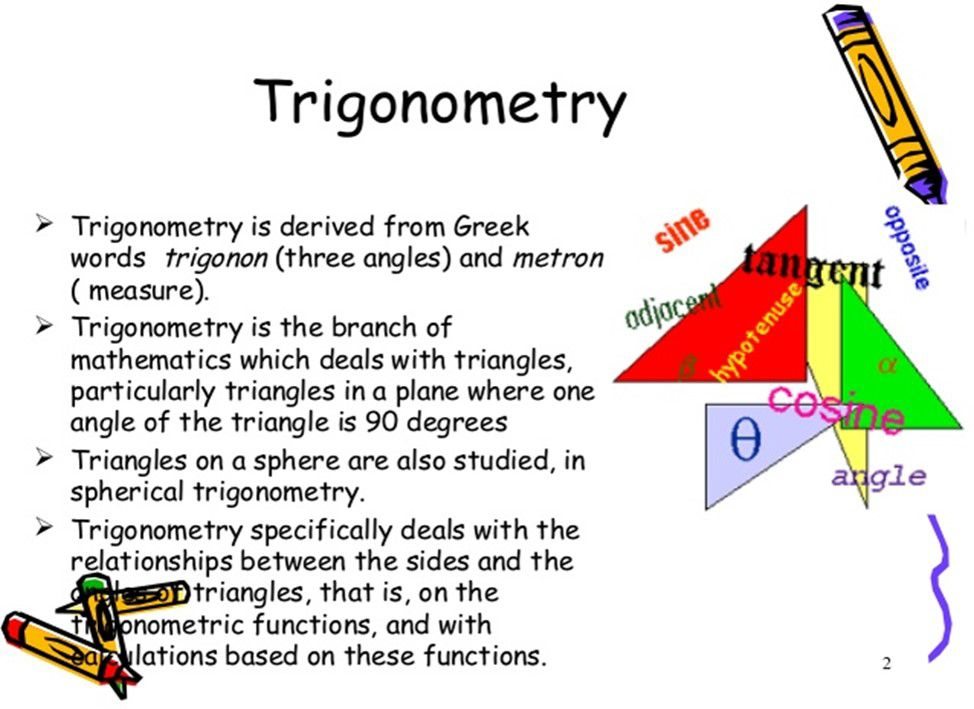Trigonometric Ratios

Here in any right angle triangle we define 6 trigonometric ratios which are Sine, Cosine, Tangent, Secant, Cosecant, and Cotangent.

So, the trigonometric ratios of an acute angle in a right triangle express the relationship between the angle and the length of its sides.

For any Triangle ABC we define the trignometric ratios as: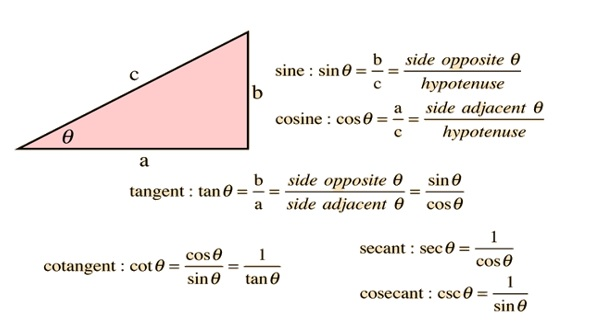Example 1: Find the value of all trigonometric ratios at angle A, if in any triangle ABC right angled at B AB=24, BC=7.

Solution: For any right angled triangle
using Pythagoras theorem we get

AB2+BC2=AC2

242+72= AC2 and hence AC =25.

Therefore from the figure

Sin A = 7/25 Cosec A = 25/7

Cos A = 24/25 Sec A = 25/24

Tan A = 7/24 Cot A = 24/7

Trigonometric Ratios for some specific Angles

If we take a triangle ABC and then if we assign the values of

When we standardize the value of θ with some of the values then we get some results which are very helpful for us and are shown in the following table: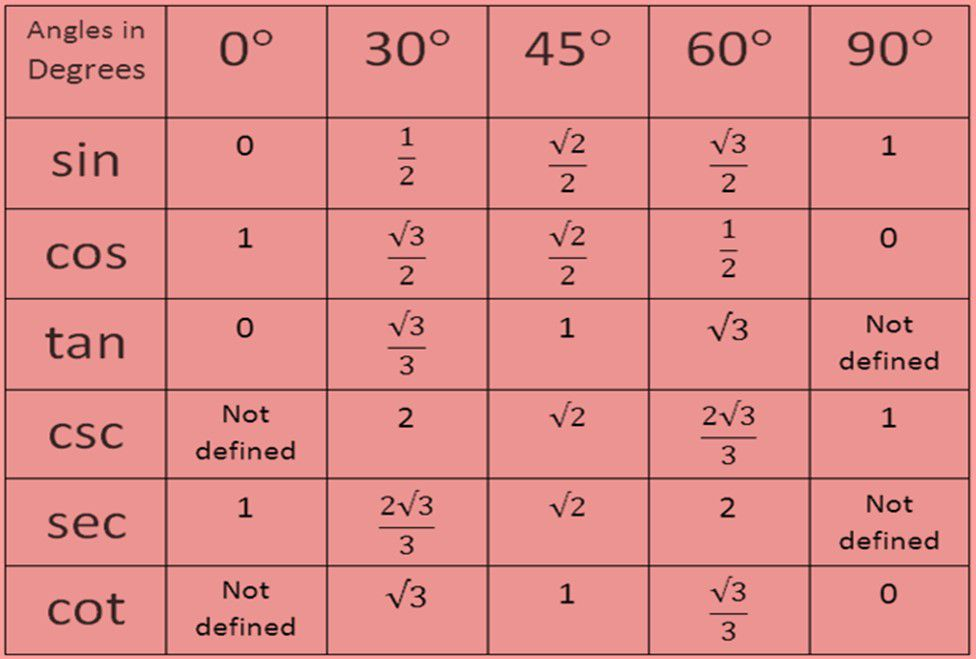Example 2: If the value of Tan (A+B) = √3 and the value of Tan(A-B) = 1/√3. And 0°

Solution: As we know that Tan 60° = √3 and Tan 30°= 1/√3.

Hence we can conclude then that:

A+B = 60° and A-B = 30° and on solving we get

A = 45° and B =15°.

Complementary Angles

Two angles are said to be complementary when their sum is 90°. Here we will discuss the complementary angles of some trigonometric formulae’s:

1. i) Cos(90°-A) = Sin A ii) Sin(90°-A) = Cos A

iii) Tan(90° -A) = Cot A iv) Cot(90°-A) = Tan A

1. v) Sec(90°-A) = Cosec A vi) Cosec(90°-A) = Sec A

Example 3: If Tan A = Cot B then prove that A+B = 90°.

Solution: As we know that Cot B = Tan (90°-B)

Tan A = Tan (90°-B) and hence we get that

A = 90° - B

Therefore, A+B = 90°. (Hence Proved)

Trigonometric Identities

1) Cos2 A + sin2 A = 1

2) 1 + tan2 A = sec2A for 0°≤A≤90°

3) Cot2 A +1 = cosec2 A for 0°≤A≤90°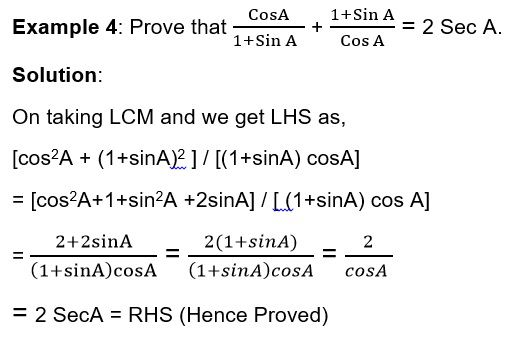Applications

If we study more about the history of the evolution and birth of trigonometry we get so many proofs of the existence of the uses of angles and thus the trigonometry involved. The basic and the most historic example is the Pyramids in Egypt.

Another big and bold example is of Theodolites which is based on the principle of trigonometry itself. Theodolite is an instruments used by civil engineers for the purpose of surveying.

The major use of trigonometry which we will study is to find the height and distance between two distant objects and we will study some basic points of how we locate ships from Light houses and a lot more.

Let’s learn some basic terminologies which we are going to discuss:

Line of Sight: The line of sight is drawn from the eye of an observer to the point in the object viewed by the observer.

Angle of Elevation: The angle of elevation of an object viewed, is the angle formed by the line of sight with the horizontal when it is above the horizontal level, i.e., the case when we raise our head to look at the object.

Angle of Depression: The angle of depression of an object viewed, is the angle formed by the line of sight with the horizontal when it is below the horizontal level, i.e., the case when we lower

our head to look at the object.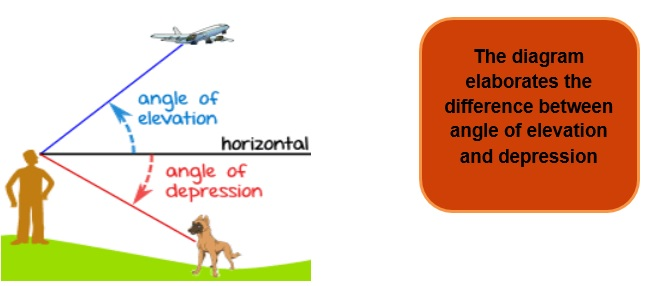Practice Questions

Q1) Prove that (1-cos2A) cosec2A = 1.

Q2) Prove that (1+cosA)(1-cosA)(1+cos2A) = 1.

Q3) Prove that (1+tan2A)(1+cot2A) (sin2A-sin4A) = 1.

Q4) State True or False:

1. a) sin A = cos(90°-A)
2. b) sin2A + 1 = cos2
3. c) tan2A + 1= sec2

Recap

• In trigonometry we deal with all the angles, lengths and various measurements using some ratios called as trigonometric ratios.
• The important identities are

1) Cos2 A + sin2 A = 1

2) 1 + tan2 A = sec2A for 0°≤A≤90°

3) Cot2 A +1 = cosec2 A for 0°≤A≤90°

• The major application part of trigonometry can be used for heights and distance for distant objects using the concept of the angle of depression and angle of elevation.

## Quiz for Trigonometry

 Q.1 There are two poles A and B, just opposite to each other, one each on either bank of a river. The height of pole A is 60 meters. From the top of pole A, the angles of depression of the top and the foot of the pole B are 30° and 60° respectively. What are the height of the pole B and the width of the river ? a) Height= 40 m Width = 34.6 m b) Height = 34.6 m Width = 40 m c) Height = 44.6 m Width = 30 m d) Height = 30 m Width = 44.6 m
 Q.2 There is a lighthouse opposite to a tower of height 40 m. The angle of elevation of the top of the lighthouse from the top of the tower is 30° and it is 60° from the foot of the tower. Calculate the height of the lighthouse. a) 20 m b) 40 m c) 60 m d) 80 m
 Q.3 There is a lighthouse opposite to a tower of height 40 m. The angle of elevation of the top of the lighthouse from the top of the tower is 30° and it is 60° from the foot of the tower. Find the distance from the foot of the tower to the top of the lighthouse. a) 40 m b) 89.7 m c) 71.4 m d) 69.2 m
 Q.4 A tower is 100 m high. Consider a point 100m from its foot. What is the angle of elevation of the top of the tower from that point? a) 0° b) 30° c) 45° d) 60°
 Q.5 There is a ladder, which is leaning against a vertical wall. The ladder is Inclined at an angle 45° to the horizontal. Now, the ladder is moved 2 m towards the wall and so , the ladder makes an angle of 60° with the horizontal now. So, what is the vertical distance moved by the ladder? a) 1.2 m b) 2.2 m c) 3.2 m d) 0.2 m
 Q.6 There is a cliff of height 150 m. A person is rowing a boat away from this cliff. At t = 0, the angle of depression at the top of the cliff is 60°. This angle changes to 45° in t = 2 minutes. What is the speed of the boat in metres per hour? a) 3499.42 b) 4499.42 c) 5499.42 d) 6499.42
 Q.7 A person is 1.5m tall. Assume that this height is measured from his foot to his eyes. He is standing 28.5 m away from a tower. The height of the tower is 30m. What is the angle of elevation of the top of the tower from the eye of the person? a) 11.56 m b) 13.48 m c) 19.78 m d) 15.46 m
 Q.8 An artist performing in a circus climbs from the ground, along a rope, stretched from the top of a vertical pole and tied at the ground. The height of the pole is 12m and the angle made b the rope with the ground level is 30°. What is the distance covered by the artist in climbing to the top of the pole? a) 20m b) 22 m c) 24 m d) 26 m
 Q.9 Consider two points A and B separated vertically by 40m, A being on the ground and B being vertically above A. There is a tower at some horizontal distance from the points. The angle of elevation of the top of the tower from A is 60° and from B is 45°. Calculate the height of the tower. a) 82.94 m b) 76.68 m c) 84.64 m d) 94.64 m
 Q.10 Two boats are moving in the middle of a sea, moving towards a lighthouse from the opposite directions. The angle of elevation from the top of the light house from the two boats are 30° and 45°. The distance between the two boats at t=0 is 100 m. Find the height of the light house. a) 54.49 m b) 45.77 m c) 49.27 m d) 40.09 m